# tutorials

## Handling IP Addresses in Machine Learning Datasets

As I was working on a small research project that employs a typical cybersecurity dataset, I came across this question. How should we handle IP addresses in these datasets? Here are my thoughts about this.

Many datasets used in research conducted in cybersecurity contain IP addresses, such as source IP address, and/or destination IP address. In most of these datasets, IPv4 addresses are expressed in the usual dotted decimal format (ex 192.168.0.1). Most programming languages, like Python, will go crazy when they see a number with three decimal points and hence the question; how do we handle them?

A question that I would like to pause before addressing the IP address handling problem; how useful are IP addresses in training models?

If you’re training a model to detect intrusions, what impact does the source IP address has on your model’s decision to classify the packet/stream as malicious? The source IP address of a single packet might not be of great help to recognize the packet as malicious. However, if that is combined with other packets maybe coming from the same source, there might be some value in knowing the source IP address. In a similar conclusion, the destination IP address of a single packet might not help in the proper classification process, but combined with packets coming from many other sources to the same destination might flag a DDoS attack. This, makes classical classification algorithms incapable of properly classifying traffic based on features relevant to a single packet.

There are other scenarios where some or all the IP addresses in the dataset do not really contribute to the classification process. On the contrary, these addresses can confuse the classifier and through the results into the wrong class. In a dataset that contains IP addresses

## Handling them as a String (really??)

If you choose to handle them in your model as strings, then their actual contribution to the training of your model is close to nothing. I advise highly against this because it doesn’t help the classification process by any means.

## Handling them as Decimal numbers

Handling IP addresses as numbers can take one of the following two paths:

### Path 1

Convert the IP address to a binary numbers, and then convert the binary number to an integer. IP addresses can easily be converted into binary numbers from their original dotted-decimal format as each octet can be converted into an 8-bit binary representation. Then, you combine these 4 8-bit octets into a single 32-bit number, and covert that to a decimal number.

The code below shows a way of doing this in python:

Assuming that the IP addresses are held in a list named “array”,

```for pnt in range(len(array)):
x = array[pnt]
z = 0
parts = x.split('.')
z = (int(parts) << 24) + (int(parts) << 16) + (int(parts) << 8) + int(parts)
array[pnt]= z```

Keeping in mind that in most cases, the IP address itself is not an indicator of an attack, and most of the time it doesn’t hold a value in the detection process. However, as we explained earlier, the relationship between different IP addresses might help the classification process for some types of attacks. The relationship between IP addresses might indicate whether the attacker is an internal actor, or an external actor as well.  With that in mind, this method maintains this relationship between IP addresses, as the system would be able to learn that IP addresses that are close to each other can be within the same network. This can help in recognizing attacks coming from botnets within the same IP cluster, for example.

### Path 2

In this path, we strip the dots from the dotted-decimal and simply add zeros to the left to complete each octet to exactly three digits. This means that the IP address 192.168.1.14 becomes 192168001014. This python code shows how this can be done

```for pnt in range(len(array)):
x = array[pnt, 0]
z = ''
parts = x.split('.')
for prt in range(3)
if len(parts[prt])=3:
z = parts[prt] + z
elif len(parts[prt]=2:
z = '0'+ parts[prt] + z
else:
z = '00' + parts[prt] + z
array[pnt]= int(z)```

This method also maintains the relationship between IP addresses and helps the recognition of adjacent nodes and networks.

## One Hot Encoding

In this method, IP addresses get encoded into representative codes instead of being dealt with as values (whether string, or integer ones). When using this method, each IP address would be represented as a code (such as a binary code). This way, you can identify each packet coming from, or going to, a particular IP address. However, this representation will eliminate the relationship between these IP addresses. This means that your system would not be able to identify the relationship between IP addresses of two hosts within the same network.

Although the relationship is not maintained between IP addresses in this representation, it can be helpful in recognizing data flows, and doing statistics analysis of data originating or landing in a specific node. Even if the system doesn’t know what the exact IP address in a packet, it can easily calculate the amount of traffic this node is sending or receiving, or the amount of data going from a particular source to a particular destination.

For example, if your dataset contains 110 unique IP addresses, these will be replaced with 7-bit codes. The first IP address appearing in the dataset would be name ‘0000000’. Consequently, and mention of the same IP address will be encoded as ‘0000000’. The second IP address, and all of it’s consequent mentions, would be replaced with ‘0000001’ and so on.

In python this can be done through the use of SKLearn Label Binarizer.

## Final Remarks

As we discussed earlier, IP addresses are not always helpful to include within the training process. In any way you choose to represent them, they can hinder the system’s learning and have a damaging impact to the system’s outcome. A general rule of thumb is that do not include IP addresses in your training data unless you recognize a tangible benefit from it. If you’re implementing a system to detect denial of service attacks, IP addresses can help. If your system needs to differentiate internal from external traffic, you need to include IP addresses. Just look at how the IP address impacts the attack detection process and make a decision.

If you’ve used other ways of handling IP addresses, I’d love to hear from you. Perhaps, I’d include it in this article as an update. Let me know what you think in the comments section below, or shoot me an email.

## Subnetting with Variable-Length Subnet Mask (VLSM)

In this tutorial, we will go through steps of subnetting a network address using multiple subnet masks. If you’re looking for classful subnetting, you checkout my other tutorial here. After the detailed steps, we will go through two completely solved example and three more unsolved examples for you to solve. Grab a pencil and a piece of paper and let’s go.

To start with, we will assume that you have a specific number of subnets with a specific number of hosts in each subnet. What we will do in the process of subnetting is identifying the subnet address, subnet mask, subnet broadcast address, range of available host addresses for each subnet.

Subnetting generally means using some bits of the host address to generate subnet address.

Subnetting Steps:

Step 1: Choosing the Network Address

If you were given a specific network address to use in subnetting, jump to step 2. If it is up to you to choose the network address and IP address class, you may select it based on the following steps:

1. For each subnet calculate a BlockSize.
2. The BlockSize is calculated by taking the No. of Hosts+2 and rounding it to the next power of 2 (ex: 2, 4, 8, 16, 32, 64..etc which is actually 21, 22, 23, 24, 25,..etc). When we say “round to the next”, it means that 17 is rounded to 32 and 5 is rounded to 8. The result of rounding is the BlockSize. Now we have the BlockSize for each subnet.

Note: We put +2 because the subnet address and the broadcast address cannot be used by hosts.

1. Add all BlockSize for all subnets. If the sum is 256 or less, you can go for Class C address. If the result is higher than 28(which equals 256) but less than 216 (which equals 65,536), go for Class B. If the result is more than 216, go for Class A.
2. Since subnetting mostly happens for LANs, the addresses that we will use are in the Private ranges of each class as shown in the table:
 Address Class Private Range of IP addresses C 192.168.0.0 – 192.168.255.255 B 172.16.0.0 – 172.16.255.255 A 10.0.0.0 – 10.255.255.255

For the sake of simplicity, we usually choose 192.168.0.0 for Class C, and 172.16.0.0 for Class B.

Step 2: Choosing the Subnet Mask for Each Subnet

The calculation is really simple. Using the BlockSize that you have calculated in Step 1, you can find the number of bits required for host address after subnetting (Lets call it HBits) by the following calculation:

BlockSize=2HBits

This means that only HBits number of bits will be used for host addresses and the rest of the bits will be used for subnet addresses.

From that, you can easily calculate the number of bits used for subnet address (lets call it NBits) by:

NBits=32-HBits

Now you can produce the subnet mask by starting (from the left) with NBits ones and HBits zeros afterwards.

For example, if NBits=20 and HBits=12

1111 1111.1111 1111.1111 0000.0000 0000

255.255.240.0

Repeat this step for each subnet.

Step 3: Filling-up the Table

Now that we know the subnet mask, we will need to find the rest of the parameters (subnet address, broadcast address,…etc)

 S/N No S/N Address S/N Mask Broadcast Address Range of Host Addresses 0 1 2 …
1. Calculating the Subnet Address:

The first thing to do is to re-order subnets in according to their BlockSize in descending order. This is extremely important.

The first subnet address is usually the main network address. Next subnet address can be easily calculated by adding 1 to the network part of the previous subnet address. Remember to do the addition in binary, so that carry bits can be pushed to the next octet (8-bits) on the left.

For example:

The first subnet 192.168.0.0 and the subnet mask is 255.255.255.240. In binary:

 Subnet Mask for Subnet 0 (255.255.255.240) 1111 1111 . 1111 1111 . 1111 1111 . 1111       0000 Network (and also the address of Subnet 0, 192.168.0.0) Host 1100 0000 . 1010 1000 . 0000 0000 . 0000 0000 Subnet Mask for Subnet 1 (255.255.255.248) 1111 1111 . 1111 1111 . 1111 1111 . 1111       1000 Subnet 1 (192.168.0.16) Host 1100 0000 . 1010 1000 . 0000 0000 . 0001  0 000 Subnet 2 (192.168.0.24) Host 1100 0000 . 1010 1000 . 0000 0000 . 0001   1 000 Subnet 3, 4, 5,….

You can see in the table that the subnet addresses are calculated by adding 1 to the network-part of the previous subnet address.

1. Subnet mask for each subnet was earlier calculated in Step 2.
2. Broadcast address of each subnet is the last address before the next subnet. In follow-up of the previous example in 1, if subnet 0 address is 192.168.0.0 and subnet 1 is 192.168.0.16, then the broadcast address of subnet 0 is 192.168.0.15.
3. Usable host range is simply all addresses between the subnet address and the broadcast address. Again following-up on the previous example in 1, and 2, for subnet 0 the subnet address is 192.168.0.0 and broadcast address 192.168.0.15, then the usable host range is 192.168.0.1-192.168.0.14

Example 1:

You have been assigned the duty of subnetting a network for an organization’s branch office. The headquarter’s IT manager said that you need to use the network address 192.168.12.0 with the default subnet mask (255.255.255.0). The number of hosts is shown in the diagram below.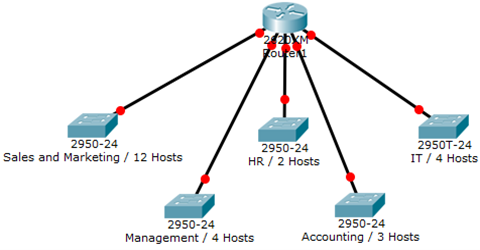Solution:

Since the main network address is already selected by the main office, we will directly start calculating the block size

HiHost=12

BlockSize= 12+2 rounded-up to the nearest power of 2.

BlockSize=16

Now we calculate the resulting subnet mask:

2HBits=16  ® 2HBits=24 ® HBits=4

NBits=32-4=28

Thus,

Subnet Mask is 1111 1111.1111 1111.1111 1111.1111 0000 ® 255.255.255.240

Now we start calculating subnets

 Subnet Mask (255.255.255.240) 1111 1111 . 1111 1111 . 1111 1111 . 1111       0000 Network (and also the address of subnet 0, 192.168.12.0) Host 1100 0000 . 1010 1000 . 0000 1100 . 0000 0000 Subnet 1 (192.168.12.16) 1100 0000 . 1010 1000 . 0000 1100 . 0001 0000 Subnet 2 (192.168.12.32) 1100 0000 . 1010 1000 . 0000 1100 . 0010 0000 Subnet 3 (192.168.12.48) 1100 0000 . 1010 1000 . 0000 1100 . 0011 0000 Subnet 4 (192.168.12.64) 1100 0000 . 1010 1000 . 0000 1100 . 0100 0000

You can clearly notice the gradual increment in the subnet address within its first part on the right while host bits remain zero all the time.

Now we start filling the table:

 S/N No S/N Address S/N Mask Broadcast Address Range of Host Addresses 0 192.168.12.0 255.255.255.240 192.168.12.15 192.168.12.1 – 192.168.12.14 1 192.168.12.16 255.255.255.240 192.168.12.31 192.168.12.17 – 192.168.12.30 2 192.168.12.32 255.255.255.240 192.168.12.47 192.168.12.33 – 192.168.12.46 3 192.168.12.48 255.255.255.240 192.168.12.63 192.168.12.49 – 192.168.12.62 4 192.168.12.64 255.255.255.240 192.168.12.79 192.168.12.65 – 192.168.12.78

First we start inserting the Subnet addresses, and the subnet mask. Then we start using the last address in each subnet as the broadcast address. Last column to fill would be the range of host addresses which will start from the first address after the subnet address to the last address before the broadcast address.

Example 2:

You have been assigned the duty of subnetting a network for an organization. The choice of class and network address is up to you. Take into consideration the host numbers mentioned in the diagram below.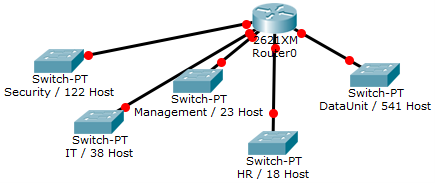Solution:

In order to make a proper network address and class choice, let us calculate the BlockSize first.

HiHost=541 ® BlockSize=541+2 rounded-up to the nearest power of two= 1024

Since the BlockSize is higher than 28 and lower than 216, we will choose class B

Let the network address be 172.16.0.0 and the default subnet mask be 255.255.0.0

Now we will calculate the subnet mask after subnetting,

BlockSize=2HBits ® 1024=2HBits ® 210=2HBits ® HBits=10

NBits=32-10=22

The chosen subnetmask will be:

1111 1111.1111 1111.1111 1100.0000 0000 ® 255.255.252.0

Now we start calculating subnets

 Subnet Mask (255.255.252.0) 1111 1111 . 1111 1111 . 1111     11 00 . 0000       0000 Network (and also the address of subnet 0, 172.16.0.0) Host 1010 1100 . 0001 0000 . 0000     00 00 . 0000 0000 Subnet 1 (172.16.4.0) 1010 1100 . 0001 0000 . 0000     01 00 . 0000 0000 Subnet 2 (172.16.8.0) 1010 1100 . 0001 0000 . 0000    10 00 . 0000 0000 Subnet 3 (172.16.12.0) 1010 1100 . 0001 0000 . 0000    11 00 . 0000 0000 Subnet 4 (172.16.16.0) 1010 1100 . 0001 0000 0001    00 00 . 0000 0000

You can clearly notice the gradual increment in the subnet address within its first part on the right while host bits remain zero all the time.

Now we start filling the table:

 S/N No S/N Address S/N Mask Broadcast Address Range of Host Addresses 0 172.16.0.0 255.255.252.0 172.16.3.255 172.16.0.1 – 172.16.3.254 1 172.16.4.0 255.255.252.0 172.16.7.255 172.16.4.1 – 172.16.7.254 2 172.16.8.0 255.255.252.0 172.16.11.255 172.16.8.1 – 172.16.11.254 3 172.16.12.0 255.255.252.0 172.16.15.255 172.16.12.1 – 172.16.15.254 4 172.16.16.0 255.255.252.0 172.16.19.255 172.16.16.1 – 172.16.19.254

First we start inserting the Subnet addresses, and the subnet mask. Then we start using the last address in each subnet as the broadcast address. Last column to fill would be the range of host addresses which will start from the first address after the subnet address to the last address before the broadcast address.

Unsolved Examples:

1. You have been assigned the duty of subnetting a network for an organization. The choice of class and network address is up to you. Take into consideration the host numbers mentioned in the diagram below.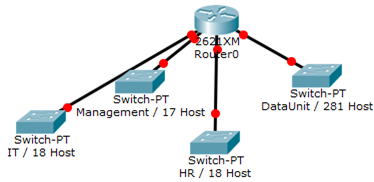1. You have been assigned the duty of subnetting a network for an organization’s branch office. The headquarter’s IT manager said that you need to use the network address 172.18.0.0 with the default subnet mask (255.255.0.0). The number of hosts is shown in the diagram below.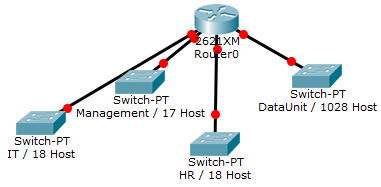1. You have been assigned the duty of subnetting a network for an organization. The choice of class and network address is up to you. Take into consideration the host numbers mentioned in the diagram below.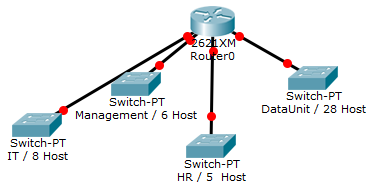## IP Subnetting Tutorial (Classful Subnetting)

This is a small subnetting tutorial that I prepared for my students back in 2015. It explains how you can “classfully” subnet an IPv4 address space. I’ll try to put together another tutorial for classless subnetting soon.

In this tutorial, we will go through steps of subnetting a network address space using a single subnet mask. After the detailed steps, we will go through two completely solved example and three more unsolved examples for you to solve. Grab a pencil and a piece of paper and let’s go.

To start with, we will assume that you have a specific number of subnets with a specific number of hosts in each subnet. What we will do in the process of subnetting is identifying the subnet address, subnet mask, subnet broadcast address, range of available host addresses for each subnet.

Subnetting generally means using some bits of the host address to generate subnet address.

Subnetting Steps:

Step 1: Choosing the Network Address

If you were given a specific network address to use in subnetting, jump to step 2. If it is up to you to choose the network address and IP address class, you may select it based on the following steps:

1. Identify the subnet with the highest number of hosts. Let’s call the highest number of hosts HiHost.
2. Round HiHost+2 to the next power of 2 (ex: 2, 4, 8, 16, 32, 64..etc which is actually 21, 22, 23, 24, 25,..etc). When we say “round to the next”, it means that 17 is rounded to 32 and 5 is rounded to 8. Let’s call the result of rounding the BlockSize.

Note: We put +2 because the subnet address and the broadcast address cannot be used by hosts.

1. Multiply the BlockSize by the number of required subnets. If the result is 256 or less, you can go for Class C address. If the result is higher than 28(which equals 256) but less than 216 (which equals 65,536), go for Class B. If the result is more than 216, go for Class A.
2. Since subnetting mostly happens for LANs within an internal network, the addresses that we will use are in the Private ranges of each class as shown in the table:
 Address Class Private Range of IP addresses C 192.168.0.0 – 192.168.255.255 B 172.16.0.0 – 172.16.255.255 A 10.0.0.0 – 10.255.255.255

For the sake of simplicity, we usually choose 192.168.0.0 for Class C, and 172.16.0.0 for Class B.

Step 2: Choosing the Subnet Mask

Since, in our subnetting, we are planning to use only one subnet mask, we can calculate it now. The calculation is really simple.

Using the BlockSize that you have calculated in Step 1, you can find the number of bits required for host address after subnetting (Lets call it HBits) by the following calculation:

BlockSize=2HBits

This means that only HBits number of bits will be used for host addresses and the rest of the bits will be used for subnet addresses.

From that, you can easily calculate the number of bits used for subnet address (lets call it NBits) by:

NBits=32-HBits

Now can produce the subnet mask by starting (from the left) with NBits ones and HBits zeros afterwards.

For example, if NBits=20 and HBits=12

1111 1111.1111 1111.1111 0000.0000 0000

255.255.240.0

Step 3: Filling-up the Table

Now that we know the subnet mask, we will need to find the rest of the parameters (subnet address, broadcast address,…etc)

 S/N No S/N Address S/N Mask Broadcast Address Range of Host Addresses 0 1 2 …
1. Calculating the Subnet Address:

The first subnet address is usually the main network address. Next subnet address can be easily calculated by adding 1 to the network part of the previous subnet address. Remember to do the addition in binary, so that carry bits can be pushed to the next octet (8-bits) on the left.

For example:

The first subnet 192.168.0.0 and the subnet mask is 255.255.255.240. In binary:

 Subnet Mask (255.255.255.240) 1111 1111 . 1111 1111 . 1111 1111 . 1111       0000 Network (and also the address of subnet 0, 192.168.0.0) Host 1100 0000 . 1010 1000 . 0000 0000 . 0000 0000 Subnet 1 (192.168.0.16) 1100 0000 . 1010 1000 . 0000 0000 . 0001 0000 Subnet 2 (192.168.0.32) 1100 0000 . 1010 1000 . 0000 0000 . 0010 0000 Subnet 3, 4, 5,….

You can see in the table that the subnet addresses are calculated by adding 1 to the network-part of the previous subnet address.

1. Subnet mask is the same calculated for all subnets from Step 2.
2. Broadcast address of each subnet is the last address before the next subnet. In follow-up of the previous example in 1, if subnet 0 address is 192.168.0.0 and subnet 1 is 192.168.0.16, then the broadcast address of subnet 0 is 192.168.0.15.
3. Usable host range is simply all addresses between the subnet address and the broadcast address. Again following-up on the previous example in 1, and 2, for subnet 0 the subnet address is 192.168.0.0 and broadcast address 192.168.0.15, then the usable host range is 192.168.0.1-192.168.0.14

Example 1:

You have been assigned the duty of subnetting a network for an organization’s branch office. The headquarter’s IT manager said that you need to use the network address 192.168.12.0 with the default subnet mask (255.255.255.0). The number of hosts is shown in the diagram below.Solution:

Since the main network address is already selected by the main office, we will directly start calculating the block size

HiHost=12

BlockSize= 12+2 rounded-up to the nearest power of 2.

BlockSize=16

Now we calculate the resulting subnet mask:

2HBits=16 → 2HBits=24 → HBits=4

NBits=32-4=28

Thus,

Subnet Mask is 1111 1111.1111 1111.1111 1111.1111 0000 → 255.255.255.240

Now we start calculating subnets

 Subnet Mask (255.255.255.240) 1111 1111 . 1111 1111 . 1111 1111 . 1111       0000 Network (and also the address of subnet 0, 192.168.12.0) Host 1100 0000 . 1010 1000 . 0000 1100 . 0000 0000 Subnet 1 (192.168.12.16) 1100 0000 . 1010 1000 . 0000 1100 . 0001 0000 Subnet 2 (192.168.12.32) 1100 0000 . 1010 1000 . 0000 1100 . 0010 0000 Subnet 3 (192.168.12.48) 1100 0000 . 1010 1000 . 0000 1100 . 0011 0000 Subnet 4 (192.168.12.64) 1100 0000 . 1010 1000 . 0000 1100 . 0100 0000

You can clearly notice the gradual increment in the subnet address within its first part on the right while host bits remain zero all the time.

Now we start filling the table:

 S/N No S/N Address S/N Mask Broadcast Address Range of Host Addresses 0 192.168.12.0 255.255.255.240 192.168.12.15 192.168.12.1 – 192.168.12.14 1 192.168.12.16 255.255.255.240 192.168.12.31 192.168.12.17 – 192.168.12.30 2 192.168.12.32 255.255.255.240 192.168.12.47 192.168.12.33 – 192.168.12.46 3 192.168.12.48 255.255.255.240 192.168.12.63 192.168.12.49 – 192.168.12.62 4 192.168.12.64 255.255.255.240 192.168.12.79 192.168.12.65 – 192.168.12.78

First we start inserting the Subnet addresses, and the subnet mask. Then we start using the last address in each subnet as the broadcast address. Last column to fill would be the range of host addresses which will start from the first address after the subnet address to the last address before the broadcast address.

Example 2:

You have been assigned the duty of subnetting a network for an organization. The choice of class and network address is up to you. Take into consideration the host numbers mentioned in the diagram below.Solution:

In order to make a proper network address and class choice, let us calculate the BlockSize first.

HiHost=541 → BlockSize=541+2 rounded-up to the nearest power of two= 1024

Since the BlockSize is higher than 28 and lower than 216, we will choose class B

Let the network address be 172.16.0.0 and the default subnet mask be 255.255.0.0

Now we will calculate the subnet mask after subnetting,

BlockSize=2HBits → 1024=2HBits → 210=2HBits ® HBits=10

NBits=32-10=22

The chosen subnetmask will be:

1111 1111.1111 1111.1111 1100.0000 0000 → 255.255.252.0

Now we start calculating subnets

 Subnet Mask (255.255.252.0) 1111 1111 . 1111 1111 . 1111     11 00 . 0000       0000 Network (and also the address of subnet 0, 172.16.0.0) Host 1010 1100 . 0001 0000 . 0000     00 00 . 0000 0000 Subnet 1 (172.16.4.0) 1010 1100 . 0001 0000 . 0000     01 00 . 0000 0000 Subnet 2 (172.16.8.0) 1010 1100 . 0001 0000 . 0000    10 00 . 0000 0000 Subnet 3 (172.16.12.0) 1010 1100 . 0001 0000 . 0000    11 00 . 0000 0000 Subnet 4 (172.16.16.0) 1010 1100 . 0001 0000 0001    00 00 . 0000 0000

You can clearly notice the gradual increment in the subnet address within its first part on the right while host bits remain zero all the time.

Now we start filling the table:

 S/N No S/N Address S/N Mask Broadcast Address Range of Host Addresses 0 172.16.0.0 255.255.252.0 172.16.3.255 172.16.0.1 – 172.16.3.254 1 172.16.4.0 255.255.252.0 172.16.7.255 172.16.4.1 – 172.16.7.254 2 172.16.8.0 255.255.252.0 172.16.11.255 172.16.8.1 – 172.16.11.254 3 172.16.12.0 255.255.252.0 172.16.15.255 172.16.12.1 – 172.16.15.254 4 172.16.16.0 255.255.252.0 172.16.19.255 172.16.16.1 – 172.16.19.254

First we start inserting the Subnet addresses, and the subnet mask. Then we start using the last address in each subnet as the broadcast address. Last column to fill would be the range of host addresses which will start from the first address after the subnet address to the last address before the broadcast address.

Unsolved Examples:

1. You have been assigned the duty of subnetting a network for an organization. The choice of class and network address is up to you. Take into consideration the host numbers mentioned in the diagram below.1. You have been assigned the duty of subnetting a network for an organization’s branch office. The headquarter’s IT manager said that you need to use the network address 172.18.0.0 with the default subnet mask (255.255.0.0). The number of hosts is shown in the diagram below.1. You have been assigned the duty of subnetting a network for an organization. The choice of class and network address is up to you. Take into consideration the host numbers mentioned in the diagram below.